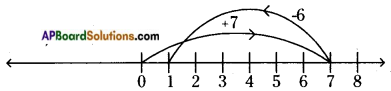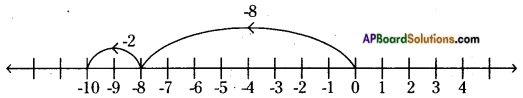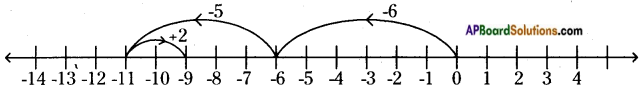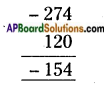# AP Board 6th Class Maths Solutions Chapter 4 Integers Ex 4.3

AP State Syllabus AP Board 6th Class Maths Solutions Chapter 4 Integers Ex 4.3 Textbook Questions and Answers.

## AP State Syllabus 6th Class Maths Solutions 4th Lesson Integers Ex 4.3

Question 1.
Add the following integers using number line.
i) 7 + (-6)
ii) (-8) + (-2)
iii) (-6) + (-5) + (+2)
i) 7 + (-6)On the number line, we first move 7 steps to the right of 0 to reach +7.
Then, we move 6 steps to the left of +7 and reach + 1.
So, 7 + (-6) = 1

ii) (-8) + (-2)On the number line, we first move 8 steps to the left of 0 to reach -8.
Then, we move 2 steps to the left of -8 and reach -10.
So,(-8) + (-2) = -10iii) (-6) + (-5) + (+2)On the number line, we first move 6 steps to the left of 0 to reach -6.
We move 5 steps to the left of -6 and reach -11.
Then, we move 2 steps to the right of -11 and reach -9.
So, (-6) + (-5) + (+2) = -9

Question 2.
(i) 10 + (-3)
(ii) 10 + (+16)
(iii) (-8) + (+8)
i) 10 + (-3)
10 + (-3) = 7 + 3 + (-3) = 7 + (3 + (-3))
= 7 + 3 – 3 = 7 + 0
∴ 10 + (-3) = +7

ii) -10 + (+16)
-10 + (+16)
= -10 + 10 + 6
= (-10 + 10) + 6
= 0 + 6
∴ -10 + (+16) = + 6

iii) (-8) + (+ 8)
-8 + (+8) = -8 + 8 = 0
∴ -8 + (+8) = 0Question 3.
Find the sum of i) 120 and -274 ii) -68 and 28
i) 120 and-274
Method -1: Sum = + 120 + (-274)
= + 120 + (- 120 – 154)
= + 120 – 120 – 154
∴ 120 + (-274) = -154

Method – II:
As the given numbers have opposite sign, we subtract one from otherAs 274 is having negative (-) sign, the answer is – 154.

ii) -68 and 28
Sum = – 68 + (28)
= – 40 – 28 + 28
∴ – 68 + 28 = – 40

Question 4.
Simplify:
i) (-6) + (-10) + 5 + 17
ii) 30 + (-30) + (-60) + (-18)